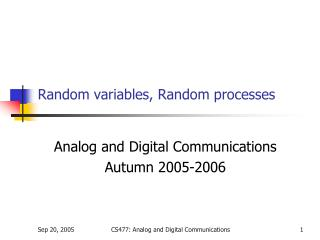DownloadDownload PresentationRandom variables, Random processes

# Random variables, Random processes

Télécharger la présentation## Random variables, Random processes

- - - - - - - - - - - - - - - - - - - - - - - - - - - E N D - - - - - - - - - - - - - - - - - - - - - - - - - - -
##### Presentation Transcript

1. Random variables, Random processes Analog and Digital Communications Autumn 2005-2006 CS477: Analog and Digital Communications

2. Random Variables • Outcomes and sample space • Random Variables • Mapping outcomes to: • Discrete numbers  Discrete RVs • Real line  Continuous RVs • Cumulative distribution function • One variable • Joint cdf CS477: Analog and Digital Communications

3. Random Variables • Probability mass function (discrete RV) • Probability density function (cont. RV) • Joint pdf of independent RVs • Mean • Variance • Characteristic function • (IFT of pdf) CS477: Analog and Digital Communications

4. Random Processes • Mapping of an outcome (of an experiment) to a range set R where R is a set of continuous functions • Denoted as or simply • For a particular outcome is a deterministic function • For or simply is a random variable CS477: Analog and Digital Communications

5. Random Processes • Mean • Autocorrelation • Autocovariance CS477: Analog and Digital Communications

6. Random Processes • Cross-correlation (Processes are orthogonal if ) • Cross-covariance CS477: Analog and Digital Communications

7. Example CS477: Analog and Digital Communications

8. Example Mean is constant and autocorrelation is dependent on CS477: Analog and Digital Communications

9. Example CS477: Analog and Digital Communications

10. Stationary and WSS RP • Stationary Random Process (RP) • Wide sense stationary (WSS) RP • Mean constant in time • Autocorrelation depends only on • Stationary  WSS (Converse not true!) CS477: Analog and Digital Communications

11. Power Spectral Density (PSD) • Defined for WSS processes • Provides power distribution as a function of frequency • Wiener-Khinchine theorem • PSD is Fourier transform of ACF CS477: Analog and Digital Communications